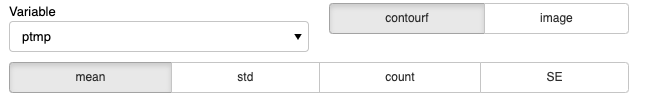# How to align two widgets in a row (a simple example)

Hi, this issue is related to this one: How do I align two widgets in a Row?

I tried to apply the solution mentioned in the issue above to align two widgets but I didnt manage to make it work. See the example panel below:I attached a reproducible example below:

``````
import panel as pn
import panel.widgets as pnw
pn.extension()

class CustomGrid(pn.GridBox):
def __init__(self, *objects, **params):
super().__init__(*objects, **params, ncols=2, nrows=2)

variables  = pnw.Select(name='Variable', value='ptmp',
options=list(variable_name))

stats_name = ('mean', 'std', 'count','SE')
options=list(stats_name))

plot_type = ['contourf','image']
types  = pnw.RadioButtonGroup(name='Type of plot', value='contourf',
options=list(plot_type))

panel_1strow = pn.Row(
pn.Param(
widgets={
'Variable': variables,
'Type of plot':types,
},
default_layout=CustomGrid,
show_name=False,
)
)

widgets1   = pn.Column(pn.Row(variables,types),stats)

widgets2   = pn.Column(panel_1strow,stats)

``````

I tried to adapt the code from the issue [How do I align two widgets in a Row?] but I didnt manage to make it work:

I am not familiar with the `pn.Param` method, the error is certainly there.
I only used `pn.Row` and `pn.Column` in a simplistic way by calling them directly on my widgets. But if I understand correctly I need to setup the default_layout parameter to solve my problem?

I don’t think you want to be using the `Param` pane here, it is meant for the situation where you have defined a `Parameterized` class and want to turn its parameters into widgets. In your example this should be fine:

``````plot_type = ['contourf','image']
types  = pnw.RadioButtonGroup(name='Type of plot', value='contourf',
options=list(plot_type), align='end')

pn.Column(pn.Row(variables,types),stats)
``````

Note the `align='end'` to align the types widget with the dropdown.

2 Likes

Thank you very much @philippjfr for the quick reply. I didn’t know about the `align` parameter!